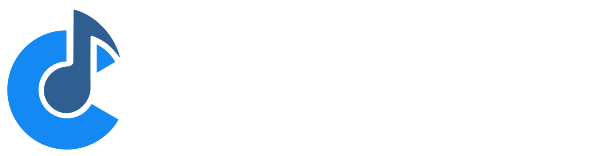# Local Variables in Controller Bar formulas

I’m struggling with the syntax for local variables (if they exist) in expressions in the Controller Bar. Local variables exist in SysEx expressions, but I can figure them out (or find info) for expressions in the Controller Bar.

I’m trying to display the currently played Note Name in the Controller bar. I have the MIDI value coming in on \$cc(32,16) and want to convert it to text - Note name + octave. I currently have:

`\$(cc(32,16)-(cc(32,16)/12)*12==0?"C":cc(32,16)-(cc(32,16)/12)*12==1?"C#":cc(32,16)-(cc(32,16)/12)*12==2?"D":cc(32,16)-(cc(32,16)/12)*12==3?"D#":cc(32,16)-(cc(32,16)/12)*12==4?"E":cc(32,16)-(cc(32,16)/12)*12==5?"F":cc(32,16)-(cc(32,16)/12)*12==6?"F#":cc(32,16)-(cc(32,16)/12)*12==7?"G":cc(32,16)-(cc(32,16)/12)*12==8?"G#":cc(32,16)-(cc(32,16)/12)*12==9?"A":cc(32,16)-(cc(32,16)/12)*12==10?"A#":cc(32,16)-(cc(32,16)/12)*12==11?"B":"X") \$((cc(32,16)/12)-1)\$("\n")\$(cc(32,16))`

… which would be a lot simpler if I could assign something like:

`var n = cc(32,16)-(cc(32,16)/12)*12`

and then (somehow) reference n as a variable.

Is there a way to do this?

BTW, I found no remainder (%) operator, and the various choices for remainder functions are not great - IEEERemainder() returns negative numbers half the time …

If you are curious why I would need to know the note I am playing - I set my Sylphyo to different keys depending on the key of the song, and then it gets transposed on some songs by MIDI Filters on various paths. When somebody asks me “what note are you playing” I have no idea (in concert pitch) what it is!

Just an idea, would it be possible to use strpart( "C;C#;D; … ", cc(32,16)-(cc(32,16)/12)*12 ), then you only had to make that calculation once and just pick the right indexed text string?

And I am not sure if divrem is supported, but worth a try.

1 Like

Couldn’t resist testing, divrem is not supported, apparently, but this one ought to do the trick:
`\$( strpart( "C;C#;D;D#;E;F;F#;G;G#;A;A#;B", cc( 32, 16 ) - ( cc( 32, 16 ) / 12 ) * 12 ) )\$( ( cc( 32, 16 ) / 12 ) - 1 )`

4 Likes

Hi @Torsten H, and hi @ClintGoss.
One question, are you speaking only single notes? or chords?
One of the buttons in my controller bar has the number of the pressed button. I should check how I set it, maybe it could be useful, maybe replacing the number with the corresponding note (Dave Dorè’s help was fundamental!!!)
I could do it later, I’m not at home right now.
Sergio

I’m doing single notes … playing a WindSynth … trying out @Torsten’s solution right now.

A-triple-Plus @TorstenH … works like a charm, less characters, less calories, the whole works!

In principle it should have been added at some point…

but I can’t get it to work, neither in Notes nor in Custom Buttons (I am on C4 4059). @TorstenH solution seems the simplest option, for now.

Gabriel

I visualised the button on my bar controller, but it is not what I thought would be useful.
The button number appears as a result of binding, I don’t think it can be useful … to the cause, it just makes the number of the button pressed visual.
Here is a photo of how this detail is set up in my Singable screen:

I hope it can be useful.
Sergio

I tried but it doesn’t work for me.
what did I miss?

in order to make it work, you have to:

1. create a route going from your keyboard to the onscreen keyboard (maybe you already have one)
2. add a “Note as controller” filter to the route, changing notes to controller number 32 and also changing MIDI channel to 16 (@ClintGoss’s formula uses CC32 on channel 16)
3. customize a button, inserting @TorstenH’s formula in the Caption field

Gabriel

Ok, I configured the setting and it works.
I put this new note button next to the fret number. It was really a nice idea and also useful!
Thank you all.
Sergio

2 Likes

Thanks for reporting. I think I added modulus to the low level expression engine used in custom curves, but forgot for the variable string expression engine. I’ll get it fixed.

3 Likes

Hi to all and Happy New Year!

I wanted to ask something on the subject that might perhaps be impractical, perhaps impossible or too complicated.
I put this button in my Bar Controller that recognises the note pressed and, as I said, it was a nice and useful thing.
But if you wanted to go further and be able to recognise a chord, do you think it could be done?
Sergio

Pianoteq has that feature build in, so in principle it is possible - but it will require a lot of programming in a script if you wanted to try to make your own for the control bar.

Hi to all!
I don’t know if this is useful.
dave_dore suggested a vst to recognise chords:

I set it up so that I have the chords on the left hand and the soloist on the rigth hand.
I thought it was nice, maybe it could be useful.

Sergio

1 Like